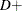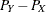# The NPAR1WAY Procedure

### PROC NPAR1WAY Statement

• PROC NPAR1WAY < options > ;

The PROC NPAR1WAY statement invokes the NPAR1WAY procedure. Optionally, it identifies the input data set and requests analyses. By default, the procedure uses the most recently created SAS data set and omits missing values from the analysis. If you do not specify any analysis options, PROC NPAR1WAY performs an analysis of variance (ANOVA option), tests for location differences (WILCOXON , MEDIAN , SAVAGE , and VW options), and empirical distribution function tests (EDF option).

Table 71.2 summarizes the options available in the PROC NPAR1WAY statement. Descriptions of the options follow in alphabetical order.

Table 71.2: PROC NPAR1WAY Statement Options

Option

Description

Input Data Set

Names the input SAS data set

Missing Values

Treats missing CLASS values as a valid class

Analyses

Requests analysis of Ansari-Bradley scores

Requests standard analysis of variance

Requests analysis of Conover scores

Requests one-sided Kolmogorov-Smirnov statistics

Requests pairwise multiple comparison analysis

Requests empirical distribution function statistics

Requests the Fligner-Policello test

Requests Hodges-Lehmann estimation for two-sample data

Requests analysis of Klotz scores

Requests analysis of median scores

Requests analysis of Mood scores

Requests analysis of Savage scores

Requests analysis of input data scores

Requests analysis of Siegel-Tukey scores

Requests analysis of Van der Waerden (normal) scores

Requests analysis of Wilcoxon scores

Analysis Details

Requests median adjustment for scale analyses

Specifies the confidence level for Hodges-Lehmann estimation

Suppresses the continuity correction for Wilcoxon

and Siegel-Tukey two-sample tests

Displayed Output

Suppresses the display of all output

Plots

Requests plots from ODS Graphics

You can specify the following options in the PROC NPAR1WAY statement.

requests an analysis of Ansari-Bradley scores. For more information, see the section Ansari-Bradley Scores. The ADJUST option subtracts class medians from the input observations before performing the analysis.

adjusts for location differences among classes before performing tests for scale differences. The ADJUST option applies to the following analyses: Ansari-Bradley (AB ), Klotz (KLOTZ ), Mood (MOOD ), and Siegel-Tukey (ST ). If you specify the ADJUST option, PROC NPAR1WAY subtracts the corresponding class median from each observation before scoring the data and performing the scale tests that you request.

You can also request adjustment for an individual scale test by specifying ADJUST in parentheses after the scale test option; for example, you can specify ST(ADJUST) to adjust by class medians before performing the Siegel-Tukey test.

ALPHA=specifies the level of the confidence limits for the Hodges-Lehmann location shift, which you can request by specifying the HL option. The value ofmust be between 0 and 1; a confidence level ofproduces% confidence limits. By default, ALPHA=0.05, which produces 95% confidence limits for the location shift.

ANOVA

requests a standard analysis of variance on the raw data.

CONOVER

requests an analysis of Conover scores. For more information, see the section Conover Scores.

CORRECT=NO

suppresses the continuity correction for the Wilcoxon two-sample test and the Siegel-Tukey two-sample test. For more information, see the section Continuity Correction.

D

requests the one-sided Kolmogorov-Smirnovandstatistics and their asymptotic p-values, in addition to the two-sided D statistic that the EDF option produces for two-sample data. The D option invokes the EDF option. If you request exact Kolmogorov-Smirnov tests by specifying the KS option in the EXACT statement for two-sample data, PROC NPAR1WAY provides D+ and D– by default. For more information about Kolmogorov-Smirnov statistics, see the section Tests Based on the Empirical Distribution Function.

DATA=SAS-data-set

names the SAS-data-set to be analyzed by PROC NPAR1WAY. If you omit the DATA= option, the procedure uses the most recently created SAS data set.

DSCF

requests the Dwass, Steel, Critchlow-Fligner multiple comparison procedure, which is based on pairwise two-sample rankings. This procedure is available for multisample data, where the number of CLASS variable levels is greater than 2. For more information, see the section Multiple Comparisons Based on Pairwise Rankings.

EDF
KS

requests statistics based on the empirical distribution function. These include the Kolmogorov-Smirnov and Cramér–von Mises tests and, if there are only two classification levels, the Kuiper test. For more information, see the section Tests Based on the Empirical Distribution Function.

The EDF option produces the Kolmogorov-Smirnov D statistic for two-sample data. You can request the one-sided D+ and D– statistics for two-sample data by specifying the D option.

FP < (REFCLASS=class-number | 'class-value')>

requests the Fligner-Policello test for two-sample data. For more information, see the section Fligner-Policello Test.

The REFCLASS= option specifies which of the two CLASS variable levels (samples) to use as the reference class X for the difference between class placement sums,. REFCLASS=1 refers to the first class that is listed in the "Fligner-Policello Placements" table, and REFCLASS=2 refers to the second class listed. The table displays class levels in the order in which they appear in the input data set. REFCLASS=class-value identifies the reference class by the formatted value of the CLASS variable. By default, the reference class is the second class listed in the "Fligner-Policello Placements" table (REFCLASS=2).

HL < (REFCLASS=class-number | 'class-value')>

requests Hodges-Lehmann estimation of the location shift for two-sample data. This option also provides asymptotic confidence limits for the location shift (which are sometimes known as Moses confidence limits). For more information, see the section Hodges-Lehmann Estimation of Location Shift. You can specify the confidence level in the ALPHA= option. By default, ALPHA=0.05, which produces 95% confidence limits for the location shift.

The REFCLASS= option specifies which of the two CLASS variable levels (samples) to use as the reference class X for the location shift, YX. REFCLASS=1 refers to the first class that is listed in the "Wilcoxon Scores" table, and REFCLASS=2 refers to the second class listed. The table displays class levels in the order in which they appear in the input data set. REFCLASS=class-value identifies the reference class by the formatted value of the CLASS variable.

By default, PROC NPAR1WAY uses the larger of the two classes as the reference class X to compute the Hodges-Lehmann location shift, YX. If both class have the same number of observations, PROC NPAR1WAY uses the class that appears second in the input data set as the reference class.

requests an analysis of Klotz scores. For more information, see the section Klotz Scores. If you specify the ADJUST option, PROC NPAR1WAY subtracts the corresponding class median from each observation before performing the analysis.

MEDIAN

requests an analysis of median scores. When there are two classification levels, this option produces the two-sample median test. When there are more than two samples, this option produces the multisample median test, which is also known as the Brown-Mood test. For more information, see the section Median Scores.

MISSING

treats missing values of the CLASS variable as a valid class level.

requests an analysis of Mood scores. For more information, see the section Mood Scores. If you specify the ADJUST option, PROC NPAR1WAY subtracts the corresponding class median from each observation before performing the analysis.

NOPRINT

suppresses the display of all output. You can use the NOPRINT option when you only want to create an output data set. This option temporarily disables the Output Delivery System (ODS). For more information, see Chapter 20: Using the Output Delivery System.

PLOTS < (global-plot-options)> < =plot-request < (plot-option)> >
PLOTS < (global-plot-options)> < =(plot-request < (plot-option)> < …plot-request < (plot-option)> > )>

controls the plots that are produced through ODS Graphics. Plot-requests specify the plots to produce, and plot-options control the appearance and content of the plots. You can specify plot-options in parentheses after a plot-request. A global-plot-option applies to all plots for which it is available, unless it is altered by a specific plot-option. You can specify global-plot-options in parentheses after the PLOTS option.

When you specify only one plot-request, you can omit the parentheses around the request. For example:

plots=all
plots=wilcoxonboxplot
plots=(wilcoxonboxplot edfplot)
plots(only)=(medianplot normalboxplot)


ODS Graphics must be enabled before plots can be requested. For example:

ods graphics on;
proc npar1way plots=wilcoxonboxplot;
variable response;
class treatment;
run;
ods graphics off;


For more information about enabling and disabling ODS Graphics, see the section Enabling and Disabling ODS Graphics in Chapter 21: Statistical Graphics Using ODS.

If ODS Graphics is enabled but you do not specify the PLOTS= option, PROC NPAR1WAY produces all plots that are associated with the analyses that you request. If you request a plot by specifying the PLOTS= option but do not request the corresponding analysis, PROC NPAR1WAY automatically invokes that analysis. For example, if you specify PLOTS=CONOVERBOXPLOT but do not also specify the CONOVER option in the PROC NPAR1WAY statement, PROC NPAR1WAY produces the Conover scores analysis in addition to the box plot.

You can suppress default plots and request specific plots by using the PLOTS(ONLY)= option; PLOTS(ONLY)=(plot-requests) produces only the plots that are specified as plot-requests. You can suppress all plots by specifying the PLOTS=NONE option. The PLOTS= option has no effect when you specify the NOPRINT option.

PROC NPAR1WAY provides box plots of scored data, median plots, and empirical distribution plots. See Figure 71.7, Output 71.1.2, Output 71.1.4, and Output 71.2.2 for examples of plots that PROC NPAR1WAY produces. For general information about ODS Graphics, see Chapter 21: Statistical Graphics Using ODS.

Global Plot Options

A global-plot-option applies to all plots for which the option is available unless it is altered by a specific plot-option. You can specify the following global-plot-options in parentheses after the PLOTS option. You cannot specify both the STATS and the NOSTATS global-plot-options in the same statement.

NOSTATS

suppresses the p-values that are displayed by default in the plots.

ONLY

suppresses the default plots and requests only the plots that are specified as plot-requests.

STATS

displays p-values in the plots. This is the default.

Plot Requests

You can specify the following plot-requests:

ABBOXPLOT
AB

requests a box plot of Ansari-Bradley scores. This plot is associated with the Ansari-Bradley analysis, which you request by specifying the AB option.

ALL

requests all plots that are associated with the specified analyses. This is the default if you do not specify the ONLY global-plot-option.

ANOVABOXPLOT
ANOVA

requests a box plot of the raw data. This plot is associated with the analysis of variance based on the raw data, which you request by specifying the ANOVA option.

CONOVERBOXPLOT
CONOVER

requests a box plot of Conover scores. This plot is associated with the Conover analysis, which you request by specifying the CONOVER option.

DATASCORESBOXPLOT
DATASCORES

requests a box plot of raw data scores. This plot is associated with the analysis that uses input data as scores, which you request by specifying the SCORES=DATA option.

EDFPLOT
EDF

requests an empirical distribution plot. This plot is associated with the analyses based on the empirical distribution function, which you request by specifying the EDF option.

FPBOXPLOT
FP

requests a box plot of Fligner-Policello placements. This plot is associated with the Fligner-Policello analysis, which you request by specifying the FP option.

KLOTZBOXPLOT
KLOTZ

requests a box plot of Klotz scores. This plot is associated with the Klotz analysis, which you request by specifying the KLOTZ option.

MEDIANPLOT
MEDIAN

requests a stacked bar chart showing the frequencies above and below the overall median. This plot is associated with the median score analysis, which you request by specifying the MEDIAN option.

MOODBOXPLOT
MOOD

requests a box plot of Mood scores. This plot is associated with the Mood analysis, which you request by specifying the MOOD option.

NONE

suppresses all plots.

SAVAGEBOXPLOT
SAVAGE

requests a box plot of Savage scores. This plot is associated with the Savage analysis, which you request by specifying the SAVAGE option.

STBOXPLOT
ST

requests a box plot of Siegel-Tukey scores. This plot is associated with the Siegel-Tukey analysis, which you request by specifying the ST option.

VWBOXPLOT
VW
NORMALBOXPLOT
NORMAL

requests a box plot of Van der Waerden (normal) scores. This plot is associated with the Van der Waerden analysis, which you request by specifying the VW (NORMAL) option.

WILCOXONBOXPLOT
WILCOXON

requests a box plot of Wilcoxon scores. This plot is associated with the Wilcoxon analysis, which you request by specifying the WILCOXON option.

Plot Options

The following plot-options are available for any plot-request. You cannot specify both the STATS and the NOSTATS plot-options for the same plot. If you specify both the NOSTATS global-plot-option and the STATS plot-option for an individual plot-request, the STATS plot-option overrides the global-plot-option and displays statistics in the individual plot.

NOSTATS

suppresses the p-values that are displayed in the plot by default.

STATS

displays p-values in the plot. This is the default.

SAVAGE

requests an analysis of Savage scores. For more information, see the section Savage Scores.

SCORES=DATA
PERM

requests an analysis that uses input data as scores. This option gives you the flexibility to construct any scores for your data with the DATA step and then analyze these scores with PROC NPAR1WAY. For more information, see the section Scores for Linear Rank and One-Way ANOVA Tests.

To produce the two-sample permutation test that is known as Pitman’s test, provide raw (unscored) data in the input data set and specify the SCORES=DATA option in the EXACT statement. For more information, see the section Exact Tests.